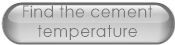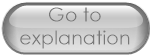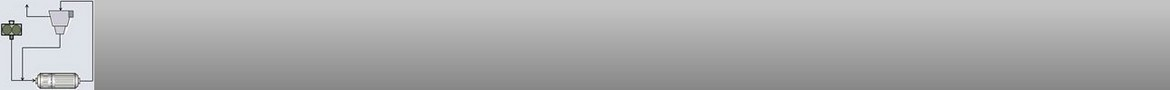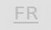The calculation of the thermical balance of the ball mill is now proposed according 2 methods:

- The first method, we know the ventilation of the mill + the cement temperature and we want to calculate the water quantity we need to inject in order to get the temperature at mill outlet we would like to have.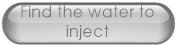- The second method, we know the ventilation inside the mill + the quantity of water injected and we calculate the temperature of the cement at mill outlet. In this calculation, the user needs to re-type the cement temperature found by the calculator in order to find the best correlation.
This procedure is necessary because the spreadsheet converter doesn't accept the SOLVER add-on.